Control Index

Control System Response

Introduction

A typical system requiring control is a tool carrier moving along a slide by the action of a DC motor which is controlled by a control which has an input set position signal and slide position signal..The characteristic of the DC motor is that the developed torque is proportional to the applied voltage.   The motor drives the carrier using a direct drive system such that the force is directly proportional to the motor torque.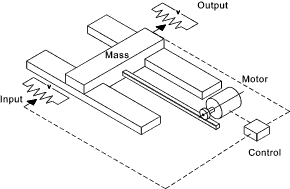The carrier has a mass (M)...  The motor indirectly produces a force on the carrier (F)....   The force from the motor is proportional to the voltage from the control F = k 1.V .....  The voltage from the control is proportional to the difference in the set position signal x i and the carrier position x o...  It is assumed that control voltage V = k 2 ( x i - x o) = k 2 .x e...

Therefore the control action of the system ...

F = k 1 .k 2.x e = k 1 .k 2 ( x o-x i )

The response of the motion of the system assuming negligible damping (Force = mass x acceleration) ...

F = M d 2x /d 2 t

In control engineering it is common to use the operator "D" in place of d/dt. ...

Therefore k 1.k 2 ( x o - x i ) = M.D 2 x o

M.dx o2 /dt 2 + k 1.k 2 x o = k 1.k 2 .x i

Using Block Diagram notation this is shown as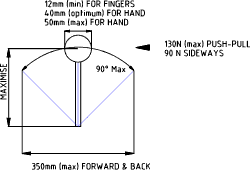System Response Definition

The total response of a linear constant differential equation is the sum of the free response and the forced response.Another way of defining the total response is as follows..

 The total response is the sum of the Steady State response and the Transient Response The Steady State Response is that part of the total response which does not approaches zero as time approaches infinity The Transient Response is that part of the total response which approaches zero as time approaches infinity

System Response

It is a primary function of control engineering to establish the response of the system to a particular input.. Considering a step input of x i = x a.. applied from time t = 0.   It can be proved that the response of the system =

x o = x a (1-cos ω n.t ) ....   By making ω n2 = (k 1k 2 )/M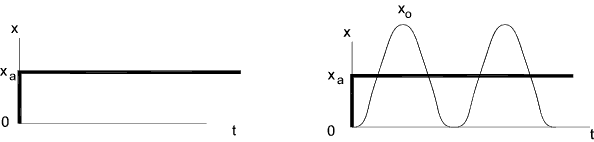Note: In reality the actual mass would not oscillate but would tend to stop due to friction forces within the system.

Assuming zero or low friction the reponse is clearly unacceptable.   The ideal response is for the system to rapidly move to the required position x a.

This can be conveniently achieved by introducing additional damping to the system.  Damping is included by attaching a viscous damper to the mass.  This damping would introduce a Force (F d ) which is proportional the velocity of the mass.   F d = - C.Dx o where C is the damping factor.

Motion with Damping

The equation of motion for the system now becomes

M.dx o2 /dt 2 + C. dx o/dt + k 1.k 2 x o = k 1.k 2 .x i

The control block diagram is modified as follows..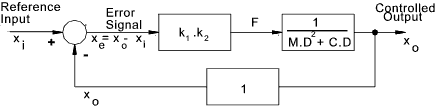Replacing k 1k 2 with K..... and using the D operator The equation of motion becomes

M.D2x o + C. D.x o + K. x o = K .x i

Dividing throughout by M and Replacing ω n2 = K / M and using the damping factor ζ = (C/2) (KM)
the equation becomes the standard equation for free vibration of a damped system.

(D2 + 2 ζ.ω n D + ω n2 ).x o = ω n2.x i

For the step input x i = x a standard solutions are obtained.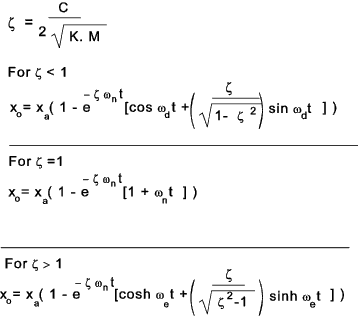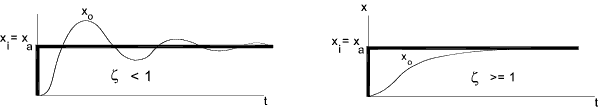Velocity Feedback

For this requirement damping provides an improvement. However viscous damping absorbs power and is accurate control using viscous damping is difficult. It is more practical to achieve the same effect by introduction of velocity feedback (C. D.x o ). This control scheme is shown below..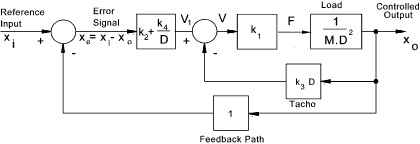The equation of motion can be derived from the block diagram as follows.
The error x e = x i - x o
The signal to the motor = V = k 2 .(x i - x o ) - k 3. D.x o
The force from the motor F = k 1 ( k 2 .(x i - x o ) - k 3. D.x o ) = k 1. k 2. x i - k 1. k 2. x o - k 1. k 3. D .x o

The resulting motion of the mass = D2.x o
The resulting motion equation D2.x o = k 1. k 2. x i - k 1. k 2. x o - k 1. k 3. D .x o
Substituting K for k 1. k 2 and C for k 1. k 3 the following recognisable equation results

M.D2x o + C. D.x o + K. x o = K .x i

The damping C coefficient can be adjusted by simply adjusting the electrical signal from the velocity feedback sensor.  This method of providing damping is called output velocity feedback.    A variation of this principle is obtained by use of Proportional + Derivative control action ( P.D )

Reducing System Errors

The system error equation includes the error terms on the LHS and the input variables on the RHS.  The error equation relating to the above system is as follows.

(M.D2 + C. D + K) x i - x e = K .x i..therefore
(M.D2+ C.D + K ) . x e = (M.D2 + C.D ). x i

If the input control requirement is a constant value i.e x a The derivatives of this with respect to time = 0.    Therefore for a constant input value the error equation becomes..

(M.D2+ C.D + K ) . x e = 0

The error value is the input value the output value ( x i - x o ) . Therefore the solution to the above equation for a constant value x a is conveniently obtained from the above solutions as follows: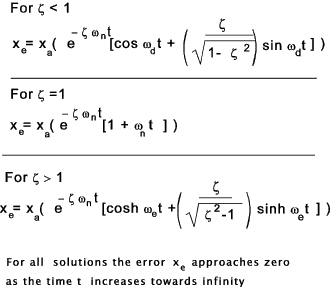The error resulting from inputs which are time related i.e ramp or sinusoidal are much more complicated.
Consider the case x i = r a.t. The RHS of the error equation becomes..

(M.D2 + C.D ). r a t = C. r a

The error equation becomes

(M.D2+ C.D + K ) . x e = C. r a

The is very similar to the equation of motion for the system with damping with x o replaced by x e and K .x i replaced by C. r a

The complete solution of the equation includes a complimentary function and a particular integral.

 The complimentary solution which is the transient component of the solution which function dies away over time if sufficient positive damping is present The particular integral or steady state solution remains after the transients have died away.

The transient component are obtained as above.
The steady state error is obtained from the basic equations with the components M.D2. x e and C.D. x e = 0 as the error is constant with respect to time.

K . x e = C. r a     Therefore     x e = (C /K ). r a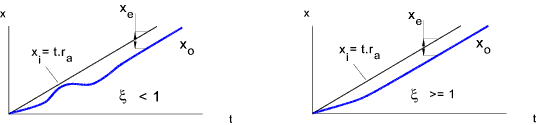Having a residual error is normally not satisfactory.   A method of eliminating this error is to include an Integral control action...This may be done by adding an integral function to the error signal conditioning unit as follows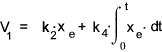In the D notation this is shown as follows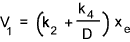The block diagram is modified accordingly as follows: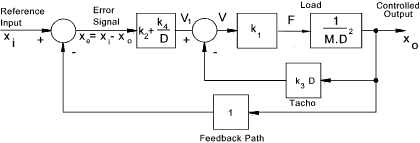The equation of motion can be derived from the block diagram as follows.
The error x e = x i -x o
The signal to the motor = V = k 2 .(x i - x o ) + (k 4 /D ) .(x i - x o ) - k 3. D.x o
The force from the motor F = k 1 [ k 2 .(x i - x o ) + (k 4 /D ) . (x i - x o ) - k 3. D.x o ]
= k 1. k 2. x i - k 1. k 2. x o + (k 1. k 4 /D ) . x i - (k 1. k 4 /D ) . x o - k 1. k 3. D .x o

The resulting motion of the mass = M. D2.x o
The resulting motion equation M.D2.x o = k 1. k 2. x i - k 1. k 2. x o + (k 1. k 4 /D ) . x i - (k 1. k 4 /D ) . x o - k 1. k 3. D .x o
Substituting K for k 1. k 2 and C for k 1. k 3 and I for k 1. k 4 the following equation results

(M.D2 + C.D + K + I/D ) x o = (K + I/D ) x i

Replacing x o with x i -x e results in the equation for error vs input x e vs x i

(M.D2 + C.D + K + I/D ) (x i - x e) = (K + I/D ) x i  therefore
(M.D2 + C.D + K + I/D ).x e = (M.D2 + C.D ) x i

Again consider the case x i = r a.t. The RHS of the error equation becomes..

(M.D2 + C.D ). r a t = C. r a

The error equation becomes

(M.D2+ C.D + K + I /D ) . x e = C. r a

To convert this to a pure differential equation it is simply differentiated through with respect to time which effectively involves multiplying x D. The RHS (C.r a ) is a constant which when differentiated with respect to time = 0..

(M.D3+ C.D2 + K.D + I ) . x e = D.C. r a = 0

The introduction of the Integral function has eliminated the constant steady state error but has raising the order of the control equation from a two to three and the result of this has been to increase the risk of stability problems...Stability is covered separately...

Control Analysis

The above outline notes introduce the general control principles in the time domain. Much of the analysis of control systems are completed by taking laplace transforms and analysing the resulting equations using root loci, Nyquist of Bode plots.. These areas of work are covered in outline on the following pages.

 Sites & Links For Control Information AB robots..Control laws:; Lots of useful control notes :; shame about the pop:;ups Software Integration..Web:;Based Control System Design and Analysis System response..Notes and examples of 1st & second order system response http://www.mash.dept.shef.ac.uk/Resources/web-partialfractions.pdf

Control Index